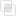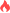php计算汉明距离总和的方法2021-07-08 16:54:51881浏览 · 0收藏 · 0评论```输入: 4, 14, 2

```class Solution {

/**

* @param Integer[] \$nums

* @return Integer

*/

function totalHammingDistance(\$nums) {

\$count = count(\$nums);

\$sum = 0;

for (\$i = 0; \$i < \$count - 1; \$i++) {

for (\$j = \$i+1; \$j < \$count; \$j++)

{

\$sum += \$this->hm(\$nums[\$i], \$nums[\$j]);

}

}

return \$sum;

}

// 汉明距离方法

function hm(\$x, \$y)

{

return substr_count(decbin(\$x ^ \$y), '1');

}}```php桥接模式的作用php安装grpc扩展的方法php引用的使用注意php引用返回如何理解php变量如何声明php变量如何命名php变量的引用赋值使用php中的四种标量类型php变量中的两种复合类型php变量有哪些特殊类型php定义常量方法的区别php常量和变量的不同php中DateTime的错误处理php中unset函数的使用php使用array_diff去除元素python unittest单元测试的过程915

python plotly如何创建滑块和选择器672

python中plotly添加交互的方法865